# Markov criterion

Jump to: navigation, search

for best integral approximation

A theorem which in some cases enables one to give effectively the polynomial and the error of best integral approximation of a function. It was established by A.A. Markov in 1898 (see ). Let,, be a system of linearly independent functions continuous on the interval, and let the continuous functionchange sign at the points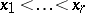inand be such that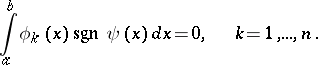If the polynomial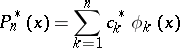has the property that the difference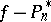changes sign at the points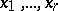, and only at those points, thenis the polynomial of best integral approximation toand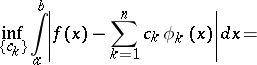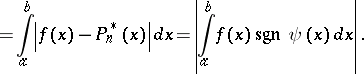For the system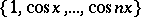on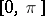,can be taken to be; for the system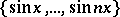,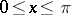,can be taken to be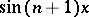; and for the system,, one can take.